Published: 20 April 2018

# An integrated network/firepower operation model based on Lanchester equation

Jin Xing Liu1
Shi Hong Xu2
Jin Song Gao3
Yi Qin Yuan4
1, 2The First Aeronautical College of Air Force, Xinyang, 464000, P. R. China
3, 4, 1Science and Technology on Electro-Optic Control Laboratory, Luoyang, 471009, P. R. China
Corresponding Author:
Shi Hong Xu
Views 103

#### Abstract

In this paper, an analysis is made to the network/firepower integrated combat mode based on the development trend of future combat equipment and existing combat cases. Then, the system dynamics model of network/firepower integrated strike is established based on the mechanism of network reconnaissance/attack and firepower attack. The Attrition-Rate Coefficients model of network/firepower combat is established by studying the effectiveness evaluation method of network attack and firepower attack. The Lanchester model of network/firepower integrated confrontation covering normal nodes, infected nodes and all infected nodes is established. In order to study the dynamic, uncertain network counter process, a type of the vibration network attack is introduced to Lanchester equation and its effectiveness in network attack process is discussed by means of the simulation results.

## 1. Introduction

In the future wars, the cooperative combat with the integration of network reconnaissance, network attacking, electronic attacking, and firepower attacking will become the main combat mode . Therefore, with the development of the equipment for network and electronic attacking, it is necessary to study the simulation and the resource planning methods of the integrated combat of network attack, electronic attack, firepower and other different types of forces, so as to use the resources reasonably [2-8]. With the arrival of information war, the classical Lanchester equation is difficult to adapt to the modern information war and meet the demand of the war. In recent years, scholars studied the Lanchester equation under the condition of information [5-10]. In the literature , the Lanchester equation of the electronic counter/fire model and force assignment algorithm is studied based on Cooperative combat of electronic warfare and fire strike force. Literature [6-8] focuses on the influence of information on operational effectiveness from the aspect of information support. Literature  makes improvement to the classic Lanchester equation and establishes the model of a network attack process. Based on the virus-spread ideas, the network attack effect on combat firepower of war between entities is studied in , which ignore the dependency of effectiveness of network attacks on network reconnaissance. In order to evaluate the network/firepower integrated attack effectiveness, in this paper, the combat mode of the network/firepower attack integrated operation is put forward, and its dynamics are given, a method for calculating the force attrition is presented, and a cooperative combat model of network /firepower attack is built based on Lanchester equation.

## 2. Integrated network/firepower attack operation modeling

### 2.1. Integrated network/firepower attack operation process

An integrated network/firepower strike situation is given in Fig. 1. The role of the Red side is executor of the integrated network /firepower attack, and that of the blue is air defense. The red force includes: network reconnaissance, network attack, aerial escort, air strike formation. The Blue includes sensor, control center, anti-aircraft weapons, and interceptor aircraft. Each combat unit of the Blue side is connected through the network. The red network reconnaissance unit receives the enemy radiation signal for recognition, positioning, and analyzing, gets the enemy command and control network topology and the weak point, makes combat decisions for network attacks and firepower attacks, and distributes the decisions to the attacking units. The network attack unit fight against the enemy network to paralyze the enemy command and control network. After the enemy network get paralysis, the firepower attacking unit will fire at the pre-assigned target. The escort airplane formation will escort the network reconnaissance and attack unit. Sensors of the Blue side detect the intrusion of the red side, and report to the allegations center, and the command center will guide ground anti-aircrafts fire and interceptor aircrafts to intercept the invasion forces of the red.

Fig. 1Network / firepower integrated combat situation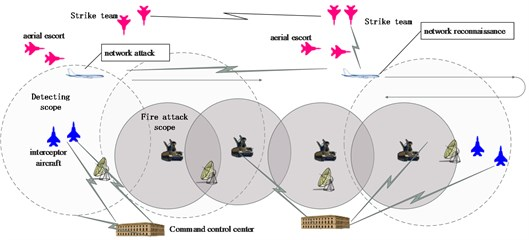### 2.2. System dynamics model

System dynamics is an influence diagram for describing mutual destruction of the two sides (Fig. 2), which is the foundation of Lanchester equation. The network attack makes soft killing to the enemy combat unit, through virus infection of the enemy software system, tampering with the command control commands, and other methods of false target deception. Soft killing cannot lead to the decrease of the number of enemy combat platforms, but can directly or indirectly lead to loss or degraded combat effectiveness of the enemy combat platform in a certain period of time or in the whole course of war.

Fig. 2System dynamics description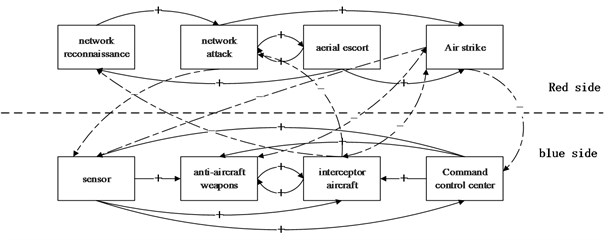### 2.3. Lanchester equation modeling

The necessary condition for launching the guided weapons is that the target is captured by sensor and enter the effective firing range. The fire between the guided weapons can be described by Lanchester square law. The implementation of a network attack is to enter an effective range of enemy wireless energy radiation and to obtain enemy information. Therefore, the Lanchester square law  is applied to describe the network operation between ourselves and the enemy.

### 2.3.1. Model of attrition-rate coefficients under network attack

In order to simplify the research, the working condition of each unit in the enemy’s command and control system after a cyber-attack is classified into three states: the normal state, the state of operational efficiency decline, and the loss of combat effectiveness. For example, after our implementation of virus attacks on the enemy’s command and control network, one of the following effects will appear: the enemy’s command and control network is not infected, or some computers in one system of the network are infected, or the entire computer system is infected, resulting in a complete paralysis of the system. Therefore, the three states are introduced to connote the states of the computers in the enemy’s command and control system after cyber-attack. After the cyber-attack, the enemy may use antivirus software to kill virus, or use the backup system to restore the computer system. Therefore, these three states can be converted to each other.

When the computer virus is injected into the command and control network, the virus can be transmitted in the command and control network. The influence of the command and control network on the enemy can be expressed in the following formula:

1
$\left\{\begin{array}{l}{\stackrel{˙}{y}}_{0}\left(t\right)=-{\lambda }_{1}{y}_{0}\left(t\right)+{\lambda }_{4}{y}_{1}\left(t\right)+{\lambda }_{6}{y}_{2}\left(t\right),\mathrm{}\mathrm{}\\ {\stackrel{˙}{y}}_{1}\left(t\right)=-{\lambda }_{3}{y}_{1}\left(t\right)+{\lambda }_{1}{y}_{0}\left(t\right)+{\lambda }_{5}{y}_{2}\left(t\right),\mathrm{}\\ {\stackrel{˙}{y}}_{2}\left(t\right)={\lambda }_{3}{y}_{1}\left(t\right)+{\lambda }_{2}{y}_{0}\left(t\right)-{\lambda }_{5}{y}_{2}\left(t\right)-{\lambda }_{6}{y}_{2}\left(t\right),\end{array}\right\$

where, ${y}_{0}$, ${y}_{1}$ and ${y}_{2}$ is the infection status of the computer, i.e. normal, partial infection, all infection; ${\lambda }_{1}$, ${\lambda }_{2}$,…, ${\lambda }_{6}$ are the transition probability of the infection state of the computers respectively.

### 2.3.2. Lanchester equation for network / firepower integrated strike

Suppose, ${x}_{d}$ is the network reconnaissance unit of the Red side, ${x}_{na}$ is the network attack unit of the Red side, ${x}_{hh}$ is the escort team, ${x}_{a}$ is the attack team; ${y}_{r}$ is the radar of the Blue side, ${y}_{aam}$ is the anti-air missile; ${y}_{lj}$ is the intercept team, ${y}_{comm}$ is the command and control unit. The Lanchester model is show as following:

2
$\left\{\begin{array}{l}{\stackrel{˙}{x}}_{d}\left(t\right)=-{a}_{lj}{\sum }_{k=0}^{k}{\mathrm{a}}_{\mathrm{i}}{y}_{ljk}\left(t\right),\mathrm{}\mathrm{}\mathrm{}\mathrm{}\mathrm{}\mathrm{}\mathrm{}\mathrm{}\mathrm{}\mathrm{}\mathrm{}\mathrm{}\mathrm{}\mathrm{}\mathrm{}\mathrm{}\mathrm{}\mathrm{}\mathrm{}\mathrm{}\mathrm{}\mathrm{}\mathrm{}\mathrm{}\mathrm{}\mathrm{}\mathrm{}\mathrm{}\mathrm{}\mathrm{}\mathrm{}\mathrm{}\mathrm{}\\ {\stackrel{˙}{x}}_{na}\left(t\right)=-{a}_{lj}{\sum }_{k=0}^{k}{\mathrm{a}}_{\mathrm{i}}{y}_{ljk}\left(t\right)-{a}_{aam}{\sum }_{j=0}^{m}{a}_{j}{{y}_{aam}}_{\mathrm{j}}\left(t\right)+Rif\left(t\right),\\ {\stackrel{˙}{x}}_{hh}\left(t\right)=-{a}_{lj}{\sum }_{k=0}^{k}{\mathrm{a}}_{\mathrm{i}}{y}_{ljk}\left(t\right)-{a}_{aam}{\sum }_{j=0}^{m}{a}_{j}{{y}_{aam}}_{\mathrm{j}}\left(t\right),\mathrm{}\mathrm{}\mathrm{}\mathrm{}\mathrm{}\mathrm{}\mathrm{}\mathrm{}\mathrm{}\mathrm{}\\ {\stackrel{˙}{x}}_{a}\left(t\right)=-{a}_{lj}{\sum }_{k=0}^{k}{\mathrm{a}}_{\mathrm{i}}{y}_{lji}\left(t\right)-{a}_{aam}{\sum }_{j=0}^{m}{a}_{j}{{y}_{aam}}_{\mathrm{j}}\left(t\right),\mathrm{}\mathrm{}\mathrm{}\mathrm{}\mathrm{}\mathrm{}\mathrm{}\mathrm{}\mathrm{}\mathrm{}\\ {\stackrel{˙}{y}}_{ri}\left(t\right)=-{b}_{hh}{d}_{i}{x}_{hh}\left(t\right)-{\sum }_{i=0}^{m}{\lambda }_{ik}{y}_{ri}\left(t\right),\mathrm{}\mathrm{}\mathrm{}\mathrm{}\mathrm{}\mathrm{}\mathrm{}\mathrm{}\mathrm{}\mathrm{}\mathrm{}\mathrm{}\mathrm{}\mathrm{}\mathrm{}\mathrm{}\mathrm{}\mathrm{}\mathrm{}\\ {\stackrel{˙}{y}}_{aamj}\left(t\right)=-{b}_{hh}{e}_{i}{x}_{hh}\left(t\right)+{\sum }_{i=0}^{m}{\lambda }_{jk}{y}_{aami}\left(t\right),\mathrm{}\mathrm{}\mathrm{}\mathrm{}\mathrm{}\mathrm{}\mathrm{}\mathrm{}\mathrm{}\mathrm{}\\ {\stackrel{˙}{y}}_{ljk}\left(t\right)=-{b}_{hh}{f}_{i}{x}_{hh}\left(t\right)+{\sum }_{k=0}^{m}{\lambda }_{kk}{y}_{ljk}\left(t\right),\mathrm{}\mathrm{}\mathrm{}\mathrm{}\mathrm{}\mathrm{}\mathrm{}\mathrm{}\mathrm{}\mathrm{}\mathrm{}\mathrm{}\mathrm{}\\ {\stackrel{˙}{y}}_{commi}\left(t\right)=-{b}_{a}{g}_{i}{x}_{a}\left(t\right)+{\sum }_{i=0}^{m}{\lambda }_{ik}{y}_{lji}\left(t\right),\mathrm{}\mathrm{}\mathrm{}\mathrm{}\mathrm{}\mathrm{}\mathrm{}\mathrm{}\mathrm{}\mathrm{}\mathrm{}\mathrm{}\mathrm{}\mathrm{}\mathrm{}\mathrm{}\mathrm{}\mathrm{}\mathrm{}\end{array}\right\$

where ${a}_{i}$ is the efficiency discount factor, represents the decline in the effectiveness of the Blue weapon system after a cyberattack; $d$, $g$, $f$, $h$ are the red factor firepower distribution of weapon systems to cyber-attacks the enemy nodes; ${\lambda }_{i}$ represents the state transfer factor for each part of the Blue weapon system after the network attack. The reinforcement force of the network attack can be described as a vibration type, and described as follow:

3
$Rif=et\mathrm{s}\mathrm{i}\mathrm{n}\left(\omega t\right),$

where $e$ is the amplitude, $t$ is the time, $\omega$ is the frequency of the vibration which is represent the uncertainty of the network.

## 3. Simulation analysis

Suppose the quantities of Red side participating platforms are respectively: network reconnaissance 20, network attack 30, escort 30, assault 50. The quantities of Blue side platforms are: sensor 80, air defense firepower 120, air interception 100, command platform 60. Before the start of the fighting, the number of virus infection of all kinds of platforms of the Blue side is 0.

### 3.1. Network reconnaissance and attack of Red side is invalid

Suppose that the Red side network reconnaissance unit fail to effectively detect the vulnerable points in the Blue command system, the Blue platforms are all in normal working condition (Fig. 3(a)). Attacked by Blue side, the units of the Red side are rapidly consumed (Fig. 3(b)).

Fig. 3Simulation results of Blue units working effectively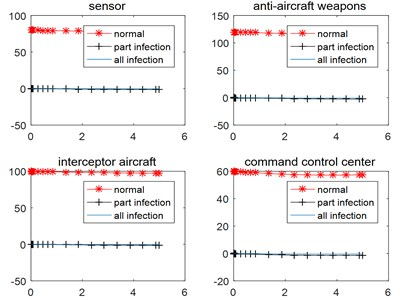a)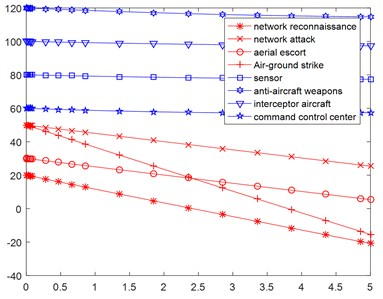b)

### 3.2. Network reconnaissance and attack of Red side is effective

Suppose that the Red side makes effective network reconnaissance and network attack, the Blue side has its nodes attacked by network, the number of normal nodes decreases, the number of partially infected and completely infected nodes increases (Fig. 4(a)), which results in decline of effectiveness of Blue sensor platform, air defense firepower, interceptor aircraft and command platform. The number of interceptors and command platforms will drop rapidly under the attack of red assault force, losing the advantage of number of battlefields (Fig. 4(b)).

### 3.3. Dynamic type network attack and the proposed reinforcement

Under the attack of the reinforcement of the network, the combat result will be changed (Fig. 5).

As in Fig. 5, the number of the normal nodes of Blue side under the vibration type network attack reinforcement, decreases faster than that of the normal network attack shown in Fig. 4, and each part of the Blue decreases to about zero within 1 second. The simulation results show that the vibration type network attack has higher effectiveness than that of the stable network attack.

Fig. 4Simulation results of no reinforcement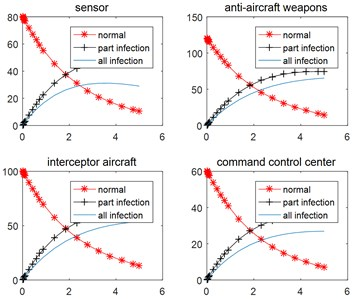a)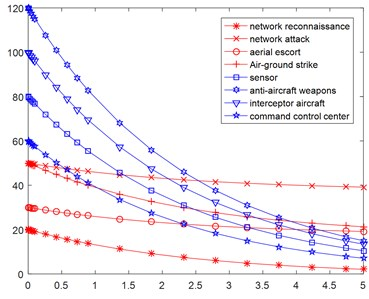b)

Fig. 5Simulation results under the vibration type of reinforcement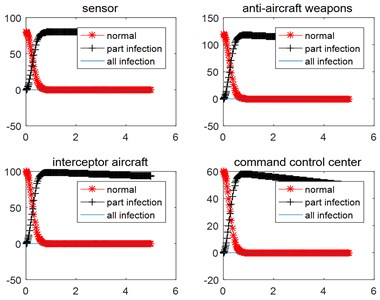a)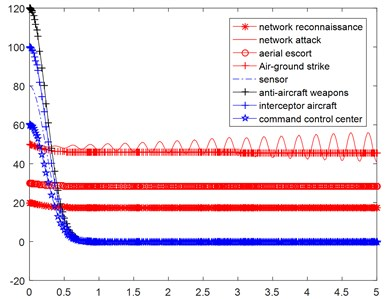b)

## 4. Conclusions

This paper focuses on the modeling of network /firepower integrated combat. The influences of partial and complete virus infection modes of network attacking on operational effectiveness of the engagement platform are described. The Lanchester equation is used as the basis for establishing the simulation model of integrated network/firepower combat. The results of this study can be used as a reference for further studies on such decision-making as effectiveness evaluation, and force assignment of the integrated network/fire combat. The simulation results and practical examples show that the uncertain network attack power has the higher effectiveness. If the vibration frequency and the amplitude are changed at the same time, the effective of the network attack and the integrated combat effectiveness will be increased further.

09 January 2018
Accepted
19 February 2018
Published
20 April 2018
SUBJECTS
Mathematical models in engineering
Keywords
network attack
firepower attack
integrated operation
Lanchester equation
performance analysis
Acknowledgements

The National Natural Science Foundation of China (No. 61672206), and Aviation Science Foundation (20165197001) support the research work.